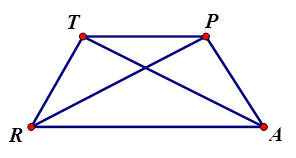# Warm-Up Trapezoids

Author:
powere
Topic:
Trapezoid

1. What are the properties of trapezoids? Check all that apply.

Select all that apply
• A
• B
• C
• D
• E

2. What are the properties of isosceles trapezoids? Check all that apply.

Select all that apply
• A
• B
• C
• D
• E
• F3. TRAP is an isosceles trapezoid where m<RTP = 75 degrees, TR = 4, PR = 7. Fill in the blank: Segment TP is _____ to segment RA.

Select all that apply
• A
• B
• C
• D

﻿4. TRAP is an isosceles trapezoid where m<RTP = 75 degrees, TR = 4, PR = 7. Fill in the blank: Segment TR is _____ to segment PA, but not _____.

Select all that apply
• A
• B
• C
• D

﻿5. TRAP is an isosceles trapezoid where m<RTP = 75 degrees, TR = 4, PR = 7. m<TRA = _____

Select all that apply
• A
• B
• C
• D

﻿﻿6. TRAP is an isosceles trapezoid where m<RTP = 75 degrees, TR = 4, PR = 7. m<RAP = _____

Select all that apply
• A
• B
• C
• D

﻿﻿7. TRAP is an isosceles trapezoid where m<RTP = 75 degrees, TR = 4, PR = 7. m<APT = _____

Select all that apply
• A
• B
• C
• D

﻿﻿8. TRAP is an isosceles trapezoid where m<RTP = 75 degrees, TR = 4, PR = 7. m<PTR = _____

Select all that apply
• A
• B
• C
• D

﻿﻿9. TRAP is an isosceles trapezoid where m<RTP = 75 degrees, TR = 4, PR = 7. PA = _____

Select all that apply
• A
• B
• C
• D

﻿﻿10. TRAP is an isosceles trapezoid where m<RTP = 75 degrees, TR = 4, PR = 7. TA = _____

Select all that apply
• A
• B
• C
• D